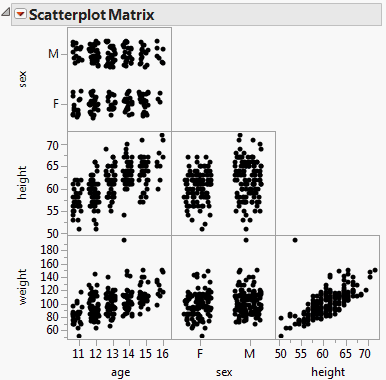Essential Graphing > Scatterplot Matrix > The Scatterplot Matrix Window
Publication date: 08/13/2020

# The Scatterplot Matrix Window

The Scatterplot Matrix window shows an ordered grouping of bivariate graphs. In each graph, you can examine the relationships between each pair of variables.

Follow the instructions in Example of a Scatterplot Matrix to produce the plot shown in Figure 6.6.